# Understanding Electrophoretic Light Scattering

Electrophoresis is the migration of macromolecules under the influence of an electric field. The electrophoretic mobility µE is defined as: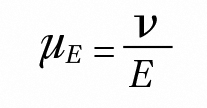(1)

where v is the particle electrophoretic velocity, and E is the applied electric field. When illuminated by a laser beam, the migrating particles cause the scattered light to be Doppler shifted, as illustrated below. The scattered light can be red- or blue-shifted depending on the direction of the particle migration. The Mobius™ measures the Doppler frequency shift Δf of the scattered light which is related to v by: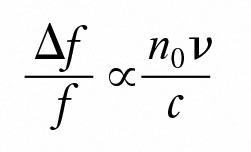(2)

where:
c is the speed of light in vacuum.
n0 is the solvent refractive index.
f is the frequency of the incident light.

Even though the Doppler shift Δf is tiny compared with the light frequency (Δf/f < 0.000000000001!), the Mobius determines the shift to high accuracy by monitoring the interference pattern between the scattered light and the reference beam in the unparalleled MP-PALS configuration. Acquiring signals at multiple angles simultaneously, MP-PALS averages away the effect of particle diffusion, producing robust measurements that are otherwise elusive when it comes to measuring the mobility of small and weakly scattering particles such as proteins.

Thanks to the independent DLS module in the Mobius, the particle hydrodynamic radius Rh can be measured simultaneously with mobility in order to compute the molecular Debye-Hückel-Henry charge ZDHHe of the particle through the relationship: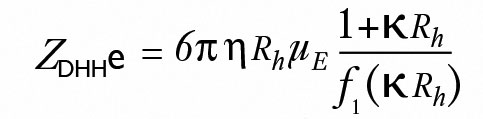(3)

where η is the solution viscosity, κ is the inverse Debye length and f1Rh) is Henry's function*.

Zeta potential, ζ, is another parameter that can be derived from the measured electrophoretic mobility. In the limiting case where the hydrodynamic radius is much larger than the thickness of the ionic double layer, Smoluchowski's equation is used: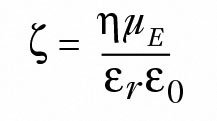(4)

where εr  is the solvent dielectric constant and ε0  the vacuum permittivity.

Alternately, when Rh is much smaller than the double layer thickness, Hückel's equation is used instead: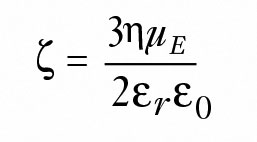(5)

References
* Tanford, C. Physical Chemistry of Macromolecules; Wiley: New York, 1961.